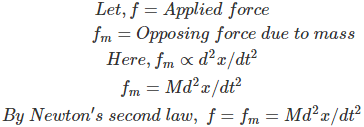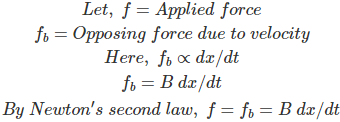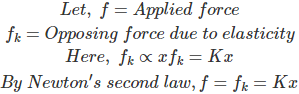# MCQs on Control Systems

##### Page 2 of 23. Go to page 1 2 3 4 5 6 7 8 9 10 11 12 13 14 15 16 17 18 19 20 21 22 23
01․ Force balancing equation of a mass element is (where, x = displacement)
M d2x/dt2
M dx/dt
M *x
any of the above

Consider an ideal mass element which has negligible friction and elasticity. Let a force be applied on it. The mass will offer an opposing force which is proportional to acceleration of the body.02․ Which of the following is/are the advantages of a closed loop control system?
The closed loop systems are accurate.
The closed loop systems are less affected by noise.
both 1 and 2.
nether 1 nor 2.

Advantages of closed loop control system: 1. The closed loop systems are accurate 2. The closed loop systems are accurate even in the presence of non linearities 3. The sensitivity of the system may be made small to make the system more stable 4. The closed loop systems are less affected by noise

03․ Force balancing equation of a dash-pot element is (where, x = displacement)
B d2x/dt2
B dx/dt
B *x
none of the above

Consider an ideal dash-pot element which has negligible mass and elasticity. Let a force be applied on it. The dash-pot will offer an opposing force which is proportional to velocity of the body.04․ Force balancing equation for elastic element (K) is (where, x = displacement)
K d2x/dt2
K dx/dt
K *x
none of the above

Consider an ideal elastic element which has negligible mass and friction. Let a force be applied on it. The elastic element will offer an opposing force which is proportional to displacement of the body.05․ Which of the following is/are the disadvantages of an open loop control system?
Inaccurate.
Unreliable.
both 1 and 2.
none of the above.

Disadvantages of open loop system: 1. Open loop systems are inaccurate and unreliable. 2. The changes in the output due to external disturbances are not correct automatically.

06․ Which of the following is the analogous quantity for mass element in force-voltage analogy?
All of the above.

The electrical equivalents of the mechanical elements in force-voltage analogy are as follows: Mechanical element ----------- Electrical analogous element
1. Mass element (M) ---------- Inductance (L)
2. Spring element (K) --------- Inverse capacitance (1/C)
3. Dash-pot (B) -------------- Resistance (R)
4. Velocity (v) ----------------- Current (i)
5. Displacement (x) ----------- Charge (q)
6. Force (f) --------------------- Voltage (V)

07․ Electrical analogous quantity for spring element (K) in force-voltage analogy is
L
R
1/C
C

The electrical equivalents of the mechanical elements in force-voltage analogy are as follows: Mechanical element ----------- Electrical analogous element
1. Mass element (M) ---------- Inductance (L)
2. Spring element (K) --------- Inverse capacitance (1/C)
3. Dash-pot (B) -------------- Resistance (R)
4. Velocity (v) ----------------- Current (i)
5. Displacement (x) ----------- Charge (q)
6. Force (f) --------------------- Voltage (V)

08․ In force-current analogy, electrical analogous quantity for displacement (x) is
flux.

The electrical equivalents of the mechanical elements in Force-Current analogy are as follows: Mechanical element ----------- Electrical analogous element
1. Mass element (M) ---------- capacitance (C)
2. Spring element (K) --------- Inverse inductance (1/L)
3. Dash-pot (B) -------------- Conductance (G=1/R)
4. Velocity (v) ----------------- Voltage (V)
5. Displacement (x) ----------- Flux (φ)
6. Force (f) --------------------- Current (i)

09․ Electrical analogous quantity for dash-pot in force-current analogy is
resistance.
conductance.
inductance.
capacitance.

The electrical equivalents of the mechanical elements in Force-Current analogy are as follows: Mechanical element ----------- Electrical analogous element
1. Mass element (M) ---------- capacitance (C)
2. Spring element (K) --------- Inverse inductance (1/L)
3. Dash-pot (B) -------------- Conductance (G=1/R)
4. Velocity (v) ----------------- Voltage (V)
5. Displacement (x) ----------- Flux (φ)
6. Force (f) --------------------- Current (i)

10․ If a circuit consists of two mass elements, two spring elements and four friction elements. Find the order of transfer function?
2
4
6
12

Order of transfer function depends only on mass elements. If a network has "n" number of mass elements, then the order of transfer function is "2×n". Therefore, from given data, number of mass elements = 2. Order of transfer function = 2×2 = 4.

<<<1234>>>